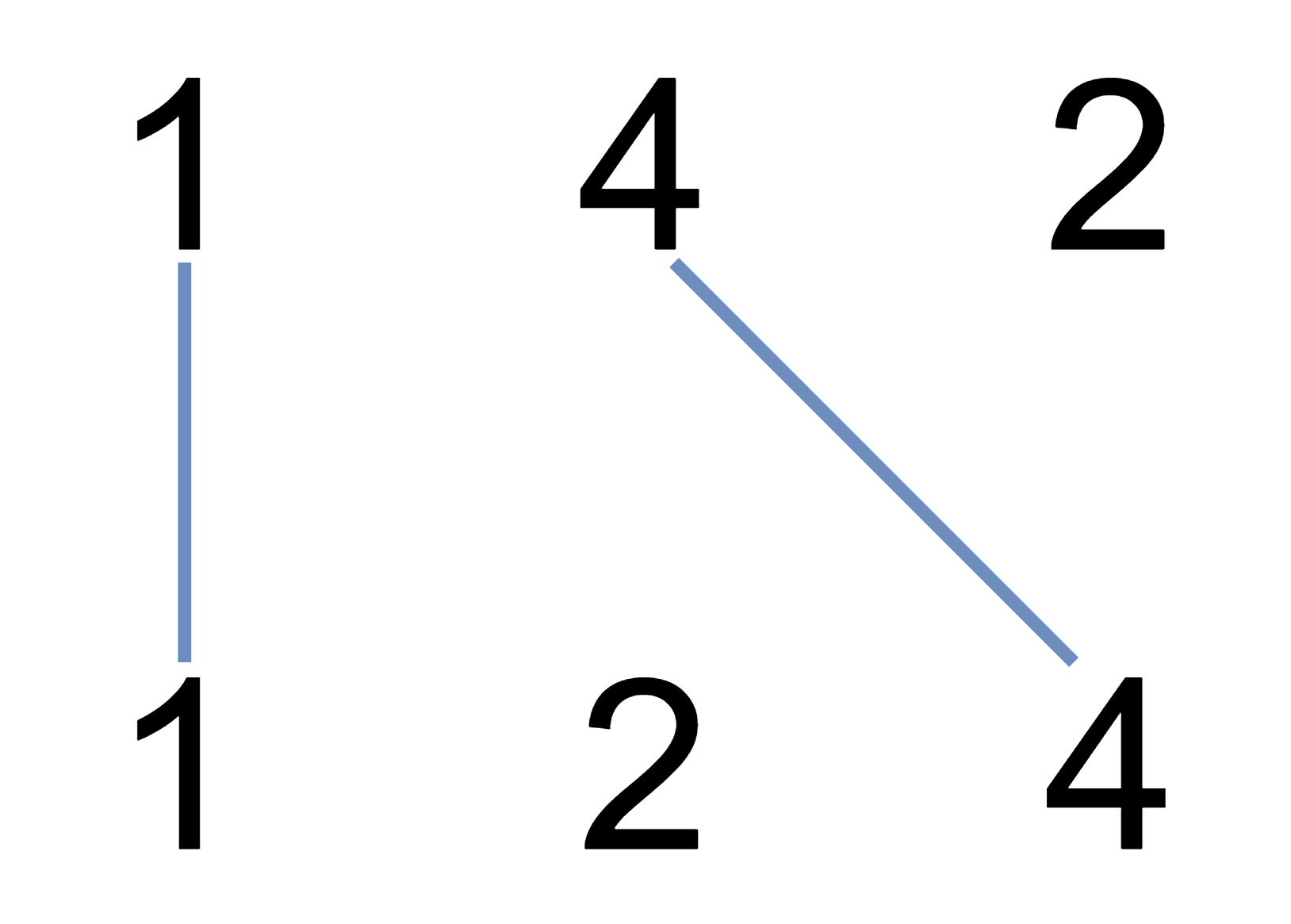# 不相交的线

## 1035. 不相交的线 (Medium)```输入：A = [1,4,2], B = [1,2,4]

```输入：A = [2,5,1,2,5], B = [10,5,2,1,5,2]

```

```输入：A = [1,3,7,1,7,5], B = [1,9,2,5,1]

1. `1 <= A.length <= 500`
2. `1 <= B.length <= 500`
3. `1 <= A[i], B[i] <= 2000`

[数组]

1. 编辑距离 (Hard)